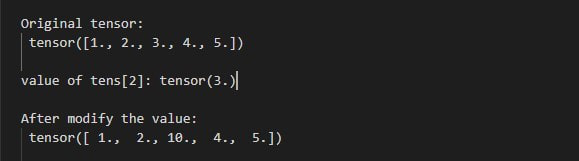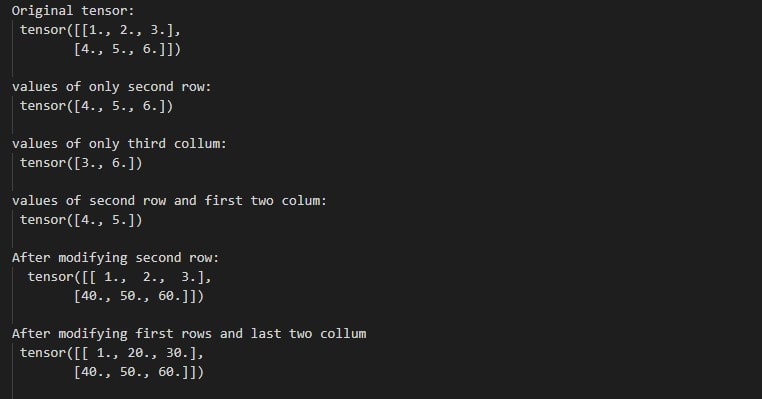# How to access and modify the values of a Tensor in PyTorch?

• Last Updated : 22 Feb, 2022

In this article, we are going to see how to access and modify the value of a tensor in PyTorch using Python.

We can access the value of a tensor by using indexing and slicing. Indexing is used to access a single value in the tensor. slicing is used to access the sequence of values in a tensor. we can modify a tensor by using the assignment operator. Assigning a new value in the tensor will modify the tensor with the new value.

Import the torch libraries and then create a PyTorch tensor. Access values of the tensor. Modify a value with a new value by using the assignment operator.

Example 1: Access and modify value using indexing. in the below example, we are accessing and modifying the value of a tensor.

## Python

 `# Import torch libraries` `import` `torch`   `# create PyTorch tensor` `tens ``=` `torch.Tensor([``1``, ``2``, ``3``, ``4``, ``5``])`   `# print tensor` `print``(``"Original tensor:"``, tens)`   `# access a value by there index` `temp ``=` `tens[``2``]` `print``(``"value of tens:"``, temp)`     `# modify a value.` `tens[``2``] ``=` `10`   `# print tensor after modify the value` `print``(``"After modify the value:"``, tens)`

Output:Example 2:  Access and modify the sequence of values in tensor using slicing.

## Python

 `# Import torch libraries` `import` `torch`   `# create PyTorch Tensor` `tens ``=` `torch.Tensor([[``1``, ``2``, ``3``], [``4``, ``5``, ``6``]])`   `# Print the tensor` `print``(``"Original tensor: "``, tens)`     `# Access all values of only second row` `# using slicing` `a ``=` `tens[``1``]` `print``(``"values of only second row: "``, a)`   `# Access all values of only third column` `b ``=` `tens[:, ``2``]` `print``(``"values of only third column: "``, b)`   `# Access values of second row and first` `# two column` `c ``=` `tens[``1``, ``0``:``2``]` `print``(``"values of second row and first two column: "``, c)`   `# Modifying all the values of second row` `tens[``1``] ``=` `torch.Tensor([``40``, ``50``, ``60``])` `print``(``"After modifying second row:  "``, tens)`   `# Modify values of first rows and last ` `# two column` `tens[``0``, ``1``:``3``] ``=` `torch.Tensor([``20``, ``30``])` `print``(``"After modifying first rows and last two column "``, tens)`

Output:My Personal Notes arrow_drop_up
Recommended Articles
Page :